# Reevaluating Amdahl's Law and Gustafson's Law

Yuan Shi

Computer and Information Sciences Department

Room 305

Temple University (MS:38-24)

shi@falcon.cis.temple.edu

215/204-6437(Voice) 215/204-5082(Fax)

October 1996

# ABSTRACT

Researchers in the parallel processing community have been using Amdahl's Law and Gustafson's Law to obtain estimated speedups as measures of parallel program potential. In 1967, Amdahl's Law was used as an argument against massively parallel processing. Since 1988 Gustafson's Law has been used to justify massively parallel processing (MPP). Interestingly, a careful analysis reveals that these two laws are in fact identical. The well publicized arguments were resulted from misunderstandings of the nature of both laws.

This paper establishes the mathematical equivalence between Amdahl's Law and Gustafson's Law. We also focus on an often neglected prerequisite to applying the Amdahl's Law: the serial and parallel programs must compute the same total number of steps for the same input. There is a class of commonly used algorithms for which this prerequisite is hard to satisfy. For these algorithms, the law can be abused. A simple rule is provided to identify these algorithms.

We conclude that the use of the "serial percentage" concept in parallel performance evaluation is misleading. It has caused nearly three decades of confusion in the parallel processing community. This confusion disappears when processing times are used in the formulations. Therefore, we suggest that time-based formulations would be the most appropriate for parallel performance evaluation.

This page is intentionally left blank to confirm with the JIDP's typesetting requirement.

1. Introduction

In parallel program evaluation Amdahl's Law has been widely cited. The analytical formulations in the literature, however, have caused much confusion to the understanding of the nature of the law . The best known misuse was perhaps the argument against massively parallel processing (MPP) .

The key to Amdahl's Law is a serial processing percentage relative to the overall program execution time using a single processor. Therefore it is independent of the number of processors. It is then possible to derive an upper bound of speedup when the number of processors (P) approaches infinity. It seemed that small serial percentages, such as 0.01-0.05, can restrict speedup to very small values. This observation had spread much pessimism in the parallel processing community. Parallel computational experiments indicate that many practical applications have indeed very small serial percentages, much smaller than we had imagined.

Gustafson revealed that it was indeed possible to achieve more than 1000 fold speedup using 1024 processors . This appeared to have "broken" the Amdahl's Law and to have justified massively parallel processing.

An alternative formulation was proposed. This is often referred to as the Gustafson's Law  and has been widely refereed to as a "scaled speedup measure". In Gustafson's formulation, a new serial percentage is defined in reference to the overall processing time using P processors. Therefore it is dependent on P. This P dependent serial percentage is easier to obtain than that in Amdahl's formulation via computational experiments. But mathematically, Gustafson's formulation cannot be directly used to observe P's impact on speedup since it contains a P dependent variable.

Unfortunately, many people have mistakenly considered the two serial percentages are identical. Gustafson's original paper contains the same error in claiming finding an exception to the Amdahl's Law..

A careful analysis reveals that these two serial percentages are directly related by a simple equation. Translating the P dependent serial percentage in Gustafson's formulation to P independent serial percentage yields an identical formula as Amdahl's. This means that there is really only one law but two different formulations. Much of the publicized arguments were indeed misunderstandings resulted from this confusion.

Another point often neglected is the prerequisite to applying Amdahl's Law. It requires the serial algorithm to retain its structure such that the same number of instructions are processed by both the serial and the parallel implementations for the same input. Often the parallel implementation is directly crafted from the corresponding serial implementation of the same algorithm.

We show that there exists a class of serial algorithms that cannot retain its structure when partitioned. Parallel programs crafted from a serial algorithm in this class can produce surprising results. For these cases, the law is open to abuse. In this paper, we provide a simple rule for identifying this class of non-structure persistent algorithms.

Finally we conclude that the use of the "serial percentage" concept in parallel program evaluation is inappropriate for it has caused much confusion in the parallel processing community for nearly three decades. This confusion disappears when the processing times are used in the formulations. Therefore we suggest that processing time based methods would be the most appropriate for parallel performance evaluation.

2. Equivalence of Gustafson's Law and Amdahl's Law

For clarity, we define the following:

ts : Processing time of the serial part of a program (using 1 processor).

tp(1) : Processing time of the parallel part of the program using 1 processor.

tp(P) : Processing time of the parallel part of the program using P processors.

T(1) : Total processing time of the program including both the serial and the parallel

parts using 1 processor =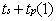.

T(P) : Total processing time of the program including both the serial and the parallel

parts using P processors =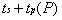.

According to the above definitions, we can further define scaled and non-scaled serial percentages as follows:

a) The scaled percentage of the serial part of the program is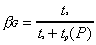and the scaled parallel part percentage is then. Note that P occurs in both percentages.

b) The non-scaled percentage of the serial part program is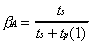and the non-scaled parallel part percentage is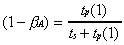. Note that P does not occur in the definitions.

It is these two definitions that are the roots of confusion.

For the Amdahl's Law (formulation) we have: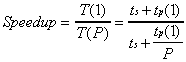(2.1)

Using the non-scaled percentages, we can reduce (2.1) to the following: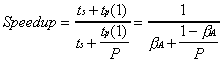(2.2)

When P approaches infinity, speedup is above bounded by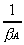. Equation (2.2) projects an unforgiving curve near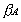=0 (Figure 1). This was the argument against using MPP systems . However, few seemed to know how to obtainpractically. This is evidenced by a widely cited technical note by Gustafson  that considersto be dependent of P.Figure 1. Predicted Speedup Using Amdahl's Law

To justify the almost linear speedup using 1024 processors Gustafson introduced a new formulation. This is often called the Gustafson's Law . This new formulation calibrates the serial percentage according to the total parallel processing time using P processors (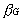):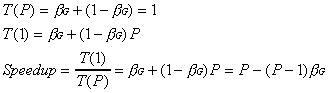(2.3)

To see the differences, let P=10, a parallel execution results=0.6, namely 40% of the elapsed time is spent on parallel processing (using 10 processors) and 60% is for sequential processing. If we putin (2.2), then Amdahl's law predicts Speedup = 10/(6.4) =1.6 while Gustafson's law gives Speedup = 10 - 5.4 = 4.6. For this reason, the speedups computed using Gustafson's formulation have been called "scaled speedups" in the literature.

This is a mistake. The problem is in the misuse ofin place ofin Amdahl's formulation. To calculatewe need to derive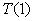. For example, let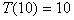seconds be the total elapsed time for the parallel algorithm that gives themeasure. The total sequential elapsed timeshould be 46 = 4 10 + 6 seconds. This yields= 6/46 = 0.13. Then the Amdahl's law gives the identical result: Speedup = 10/(1.3+0.87) = 4.6.

Mathematically, the two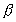's are related by a simple equation without introducing T(1):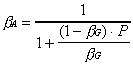(2.4)

For example, the reported serial percentages (0.4 to 0.8 percent) in the Gustafson's original paper  are really's. In order to use the Amdahl's Law correctly, we must translate's into's using (2.4). This yields= 0.0004 to 0.0008 percent respectively. Substituting these to (2.2), Amdahl's formulation predicts Speedup = 1020 to 1016 using 1024 processors.

The above discussion establishes that there is indeed only one Amdahl's Law but two different formulations. The pessimistic view of Figure 1 is still valid provided that the actual values ofis not as once thought.

3. A Class of Algorithms For Abuse

A prerequisite to applying Amdahl's or Gustafson's formulation is that the serial and parallel programs take the same number of total calculation steps for the same input. It can be very tempting to claim that the Amdahl's Law is "broken" without considering the prerequisites. In practice, however, breaking the second prerequisite may be considered "cheating" while breaking the first can be hard to avoid.

To see this, we define that a serial program is a fixed implementation of a serial algorithm. Then a parallel program is a fixed implementation of a parallel algorithm. An important characteristic of a program is that once compiled, its processing structure is fixed. Different inputs will travel different paths in the program resulting in different step counts.

There are three possible relationship between a speedup and the number of processors:

• Speedup < P, or sublinear speedup;
• Speedup = P, or linear speedup;
• Speedup > P, or superlinear speedup.

Since every practical parallel program must consolidate the final answer(s) in one program, the serial percentage in Amdahl's Law is never zero in practice. Thus, theoretically linear and superlinear speedups are not possible.

In reality, however, there are two factors that can be used to produce linear or superlinear speedups:

• Use of a resource constrained serial execution as the base for speedup calculation; and
• Use a parallel implementation that can bypass large amount of calculation steps while yield the same output of the corresponding serial algorithm.

Using the above factors, anyone can claim a "break" in Amdahl's Law by a specially engineered experiment. This was observed in a humorous note by David Bailey .

For example, an O(n2) comparison-based sort algorithm is guaranteed to "break" the law. To see this, we compare the number of worst-case algorithmic steps for sequential and parallel processing: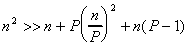, if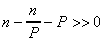. (3.1)

The left-hand-side of (3.1) represents the worst-case number of comparisons of the serial sort algorithm, right-hand-side represents the total worst-case parallel computing steps:

• There are n steps to split the input to P sorters but no comparisons are needed.
• There are P processors each doing (n/P)2 comparisons (SIMD parallelism).
• There are at most n(P-1) comparisons to merge the sorted sequences.

In (3.1), the condition is easily satisfied in practical situations. Since the worst-case communication complexity is O(n), for any processing environment, (3.1) implies there exists a problem size n such that a superlinear speedup is guaranteed.

This example illustrates a fact that the O(n2) sort algorithm cannot retain its structure when crafting a parallel algorithm from it. In other words, partitioning such a serial algorithm can improved its efficiency using only one processor.

While it is generally difficult to tell which of the "trick" factors is hidden in a speedup measure, the structure characteristics of the serial algorithm can help us to truly evaluate a parallel performance. Here we develop a simple rule to identify the algorithms that are not structure persistent.

Definition 1. A sequential algorithm is structure persistent (SP) if all parallel implementations of the same algorithm must require greater or equal number of calculation steps (including those in parallel) for all inputs.

Definition 2. A sequential algorithm is non-structure persistent (NSP) if there exists at least one parallel implementation of the same algorithm, at least one input, that the parallel implementation requires less total number of calculation steps (including those in parallel) than the total pure sequential steps.

Definition 3. A certificate is a verification algorithm that given a solution to a program it can verify the solution correctness employing a sub-algorithm of the corresponding solution algorithm.

The certificate concept was inspired by the work by Thomas Cormen, et al. . For example, the certificate for a sorting algorithm with n inputs is (n-1) pair-wise comparisons of consecutive elements. The certificate for a matrix multiplication algorithm is to multiply the matrices again. And, the certificate for an optimal Traveling Sales Person's algorithm requires solving the problem again.

Rule 1. Let the complexity of a certificate be f(n) and solution algorithm g(n), then the solution algorithm is NSP if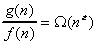, for some n>0 and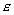> 0.

A formal proof for this rule is beyond the scope of this paper. We provide the following examples to show the vast existence of NSP algorithms.

• An O(n2) comparison-based sort algorithm is NSP, since the sort verification algorithm requires O(n), we find= 1 and n > 0. On the other hand, an O(nlgn) sort algorithm is SP.
• A linear search algorithm (O(n)) is NSP, since its certificate requires only a constant time steps. For large problem sizes, any straightforward parallel implementation can "break" the Amdahl's Law. On the other hand, a binary search algorithm is SP.
• Numerical algorithms solving f(x) = 0 type problems are NSP. This is because the certificate has a constant time complexity while the solution algorithm requires O(n) discrete steps.
• The Gauss Elimination algorithm for Ax=b type systems is NSP. This is because the certificate of such a system requires only O(n2) complexity while the Gauss Elimination algorithm requires O(n3).
• All NP-complete algorithms (solving a decision problem with exponential complexity) are NSP since their certificates are of polynomial complexity while their solution algorithms are exponential. Since both serial and parallel programs are fixed implementations of the same algorithm after compilation, there must exists an input instance such that given enough partitions, the serial program of the algorithm will take exponential number of steps while the parallel program of the same algorithm takes only polynomial steps (since there are multiple parallel sub-problems).
• NP-hard optimization algorithms are SP since we must search all states before concluding optimality. However, if an optimization algorithm use branch-and-bound heuristics, the sub-problems are NSP. This is because the sub-problems are NP-complete (solving a decision problem with exponential complexity). Therefore, it is possible for such an algorithm to "break" Amdahl's Law on selected inputs.

In general, proper partitioning of an NSP algorithm can yield more efficient algorithms. For example, the best partitioning (P value) of the above O(n2) sort algorithm can indeed produce an O(nlgn) algorithm. Re-structuring search paths in a serial program implementing a NP-complete algorithm can often lead to much better performance.

It is important to recognize that the NSP's "instruction reduction power" really should be eliminated if we want to apply Amdahl's Law correctly. This can be done by insisting on the best serial program as the basis for parallel performance evaluation. For example, for parallel sort, computing speedup using a serial program with the same partitioning factor as the number of parallel processors can eliminate superlinear speedup. For NP-complete algorithms, superlinear speedup can also be eliminated if we can force the sequential program to follow the best parallel search path. The trouble is, however, there are too many best serial programs for a given algorithm, since they are dependent on problem inputs.

It is largely inconvenient to alter a serial program whenever we add a processor (for the parallel sort) or change an input (for NP-complete algorithms) for parallel performance evaluation. We may prefer practicality over precision. Since superlinear speedup is only possible for NSP algorithms, we can detect the presence of resource factors in a performance figure if the serial algorithm is SP. This detection can help us to appreciate the value of a reported parallel performance as how much less resources are required on parallel processors as compared to a single processor.

4. Summary

This paper establishes the mathematical equivalence between the Amdahl's Law and Gustafson's Law. There is indeed only one law but two different formulations.

Using Amdahl's Law as an argument against massively parallel processing is not valid. This is becausecan be very close to zero for many practical applications. Thus very high speedups are possible using massively many processors. Gustafson's experiments are just examples of these applications.

Gustafson's formulation gives an illusion that as if P can increase indefinitely. A closer look finds that the increase inis affecting speedup negatively. The rate of speedup decrease as P approaches infinity is exactly the same as depicted by Figure 1, if we translate the scaled-percentage to a non-scaled percentage. We cannot observe the speedup impact by P using Gustafson's formulation directly since it contains a P dependent variable.

Much practical experiences have made many to "feel" the touch of the unforgiving curve in Figure 1. Perhaps this is the time to end the debate between "big-cpu" and "many-cpu" in the parallel processing community. This is because a parallel performance is dependent on many factors, such as uni-processor power, network speed, I/O system speed, problem size, problem input and lastly the serial versus parallel instruction percentages. Therefore there will never be a single solution for all problems. What we need is a practical engineering tool that can help us to identify performance critical factors for any algorithm and processing environment with systematic and practical steps. This is often called program scalability analysis.

Even though Amdahl's Law is theoretically correct, the serial percentage is not practically obtainable. For example, if the serial percentage is to be derived from computational experiments, i.e. recording the total parallel elapsed time and the parallel-only elapsed time, then it can contain all overheads, such as communication, synchronization, input/output and memory access. The law offers no help to separate these factors. On the other hand, if we obtain the serial percentage by counting the number of total serial and parallel instructions in a program, then all other overheads are excluded. However, in this case the predicted speedup may never agree with the experiments.

Furthermore, the prerequisite of having the same number of total instructions for both serial and parallel processing adds to the impracticality. This is because that for NSP algorithms the best serial program is dependent on the values of the input or the partitioning factor. Incorrect measure of the serial percentage opens many opportunities for Amdahl's Law abuse.

In the last three decades, as we have witnessed, the use of the "serial percentage" concept has caused much confusion in the parallel processing community. The confusion disappears when we start using sequential and parallel times as the basis for parallel performance evaluation. A significant additional benefit using time for parallel algorithm evaluation is that all performance critical factors can be evaluated in the same domain. Therefore program scalability analysis can be conducted for sequential and parallel programs. In comparison, it is impossible to use Amdahl's Law to conduct program scalability analysis.

## References

1. Amdahl, G.M.. Validity of single-processor approach to achieving large-scale computing capability, Proceedings of AFIPS Conference, Reston, VA. 1967. pp. 483-485.
2. Bailey, D., Twelve Ways to Fool the Masses When Giving Performance Results on Parallel Computers, RNR Technical Report, RNR-90-020, NASA Ames Research Center, 1991.
3. Corman, T. H., Leiserson, C. E., & Rivest R.L., Introduction to Algorithms, ISBN: 0-262-03141-8, MIT Press, 1990. pp. 926-932.
4. Gustafson, J.L., Reevaluating Amdahl's Law, CACM, 31(5), 1988. pp. 532-533.
5. Lewis, T.G. & El-Rewini, H., Introduction to Parallel Computing, Prentice Hall, ISBN: 0-13-498924-4,1992. pp. 32-33.

Author's Biography:

Dr. Yuan Shi earned his Master and Ph.D Degrees from University of Pennsylvania, Philadelphia, in 1983 and 1984 respectively. He is currently an Associate Professor in the CIS Department of Temple University. He is also the Interim Director for Center for Advanced Computing and Communications at Temple University since 1993, and serves as a Technical Advisory Committee member for Ben Franklin Technology Center in Philadelphia since 1993. He is the inventor of two patents in areas of heterogeneous parallel programming system and parallel computer architecture.